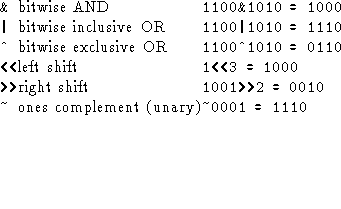# Sets

A set is data type that is useful in some data representations and algorithms. Operations on a set of objects:

• delete member

• membership

• union

• intersection

• difference

• subset

How to implement?

• As lists (use AddItem() and DeleteItem() that we previously discussed), but where we restrict an item to be on the list only once.

• As a bit vector where setting the ith bit indicates that the value of i is in the set. Makes for efficient implementation (although with a fixed number of set elements).

Pascal provides sets as a basic data type, C/C++ does not. However we can implement sets using bitwise operators available in C/C++.

## Bitwise Operators in C

With binary notation, the bits 1011 representDoing a left shift is equivalent to multiplying by 2 and doing a right shift is equivalent to multiplying by two.

Cannot actually write bit values in C, they must be octal (leading 0) or hexadecimal (leading 0x). You will see much more in computer organization.

## Set Operations Using Bitwise Operators in C

Can declare a set of integers as:

`typedef unsigned int Set;`

Make unsigned so we do not have to worry about a ``sign bit'' (again more details in computer organization).

```/*
* /cs/cs2005/pub/example/set.C -- set operations using bit operators
*/

#include <iostream.h>

#define SETSIZE (8*sizeof(unsigned int))

typedef unsigned int Set;

#define InitSet(s) s = 0;        /* initialize the set */
#define AddMember(s,i) s = s | 1<<(i) /* add a member to the set */
#define DeleteMember(s,i) s = s & ~(1<<(i)) /* delete a member from the set */
#define Member(s,i) ((s) & 1<<(i)) /* test for membership in the set */
#define Union(s1,s2) ((s1)|(s2)) /* union of two sets */
#define Intersect(s1,s2) ((s1)&(s2)) /* intersection of two sets */
#define Difference(s1,s2) ((s1)&(~(s2))) /* difference of two sets */
#define Subset(s1,s2) (((s1)&(s2))==(s2)) /* is s2 a subset of s1? */

/*
* PrintSet - print the contents of a set
*/
PrintSet(Set s)
{
int i;

for (i = 0; i < SETSIZE; i++)
if (Member(s, i))
cout << " " << i;
cout << "\n";
}

main()
{
Set s, t;

InitSet(s);
InitSet(t);
PrintSet(s);
PrintSet(t);
PrintSet(Union(s, t));
PrintSet(Intersect(s, t));
PrintSet(Difference(s, t));
DeleteMember(s, 12);
DeleteMember(s, 13);
PrintSet(s);
cout << "t " << (Subset(s,t) ? "is" : "is not") << " a subset of s\n";
DeleteMember(t, 7);
cout << "t " << (Subset(s,t) ? "is" : "is not") << " a subset of s\n";
}```

```> set
1 4 12 15
1 4 7
1 4 7 12 15
1 4
12 15
1 4 15
t is not a subset of s
t is a subset of s```

# Graphs

Only look at 10.4 in the Kruse text, Chapter 12 in Shiflet text

A graph consists of a set of vertices V and a set of edges E. Edges connect vertices and can be represented as v,w pairs where . If the pair v,w are ordered then the graph is directed, if the pair is unordered then the graph is undirected.

Examples: airline database (directed or undirected?), maps, relationships (``know'' relationship where everyone is within six connections of anyone on the planet), network routing.

A graph contains a cycle if for some point you can traverse the graph on distinct edges and return to the starting point. The graph is acyclic if it does not have a cycle. A tree is an acyclic graph.

A graph is connected if there is a path from any vertex to any other vertex.

Definitions are the same whether the graph is directed or undirected.

## Graph Representation

Often times represent a graph by indicating the set of vertices adjacent to each vertex. This representation can be formed given the set of edges for a graph.

With this representation the following implementations can be used:

• Adjacency sets - maintain an array of sets. Fig 10.14 in Kruse. Declare an array of sets ```Set rgSet[MAX]`''

• Adjacency table - two dimensional array of boolean values

`BOOLEAN Atable[MAX][MAX];`

`Atable[i][j] == TRUE` indicates that there is an edge from vertex i to vertex j. In an undirected graph only need to use half of the table.

• Adjacency lists - maintain a list of adjacent vertices (either as a linked list or contiguous storage). Look at Fig 10.15a and 10.15b from Kruse

• Mixed implementation - use an array of linked lists. Fig 10.15c from Kruse

## Graph Traversal

In many cases we want to traverse a graph. Two basic types of traversal:

1. Depth-first traversal--analagous to a preorder traversal of a tree in that we keep traversing as far away from the original node as possible. Either use recursion or keep track of nodes on a stack.

2. Breadth-first traversal--analagous to a level-by-level search in a tree. Visits all nodes adjacent to original node before moving on to the next level. Use a queue to implement.

Look at examples from text.

### Depth-First Algorithm

Maintain an array to keep track of which vertices have been visited.

Also have to check every vertex as a possible starting because the node may not be connected.

Look at algorithm in text.